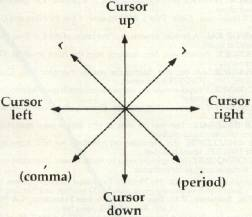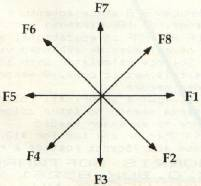`COMPUTE! ISSUE 32 / JANUARY 1983 / PAGE 213`

 VIC Pencil Ken Bowd, Huntsville, Ontario This High Resolution Drawing Program will run on a 5K VIC with 1K in reserve. When the RUN command is given, the screen will turn black (POKE 36879,8). You will have to allow a few seconds for the VIC to execute the loops. Hit the "D" key, and one pixel will light on the screen; hitting "E" will extinguish it. The pencil can be moved either continuously or one pixel at a time. The continuous movement is controlled by the function keys, while single-pixel writing is done with the "," "." "<" ">" and cursor control keys. The pencil will write left, right, up, or down, as well as in four directions diagonally. The "S" key stops all movement of the pencil. It is also possible to move the pencil without writing by hitting the "M" key. Pencil Control Single Pixel DrawingContinuous DrawingYou can clear home or clear the screen by using standard VIC operating procedures. Line 50 reserves some memory from BASIC; line 60 tells the VIC to go to RAM location 7168 for description of characters. Line 70 clears our reserved memory, and line 80 turns the screen black and clears the screen. Lines 100 through 120 set up a mini screen. Line 130 is the beginning of the main program. It asks the operator for instructions, and from this point down to line 380 the values of X and Y are assigned according to what character was "gotten" from the keyboard. Lines 390 to 420 check to make sure the pencil is on the screen. On down to line 490 the proper bit is selected, and the proper POKE is made to either turn the bit on or turn it off. Although this is really a novelty program, the kids will probably enjoy drawing on the computer. You will also find after a little practice that it is possible to draw arcs and curves. It should be noted that, although you can't draw on the entire screen, you can address 4096 different spots as compared to the usual 506. ```50 POKE56, 24 : POKE52, 24 60 POKE36869, 255 70 FOR I = 7168 TO 7679 : POKEI, 0 : NEXT 80 POKE36879, 8 : PRINTCHR\$(147) 90 FOR I = 7680 TO 8191 : POKEI, 160 : NEXT I 100 FOR L = 0 TO 7 : FORM = 0 TO 7 110 POKE7841 + M*22 + L, L*8 + M 120 NEXT : NEXT 130 GETB\$ 140 IFB\$ = "D"THENC\$ = "D" 150 IFB\$ = "E"THENC\$ = "E" 160 IFB\$ = "M"THENC\$ = "M" 170 IFB\$ = "{HOME}"THEN X = 0 : Y = 0 180 IFB\$ = "S"THENA\$ = "" 190 IFB\$ = "{Fl}"ORB\$ = "{F2}"0RB\$ = "{F3}"ORB\$ = "{F4}"ORB\$ = "{F5} "ORB\$ = "{F6}"ORB\$ = "{F7}"ORB\$ = "{F8}"THENA\$ = B\$ 200 IFB\$ = "{CLEAR}"THEN220 210 GOTO230 220 FORI = 7168 TO 7679 : POKEI, 0 : NEXT 230 IFB\$ = "{RIGHT}"THEN X = X + 1 240 IFB\$ = "{LEFT}"THEN X = X - 1 250 IFB\$ = "{UP}"THEN Y = Y - 1260 IF B\$ = "{DOWN}" THEN Y = Y + 1 270 IF B\$ = "," THEN X = X - 1 : Y = Y + 1 280 IF B\$ = "<" THEN X = X - 1 : Y = Y - 1 290 IF B\$ = "." THEN X = X + 1 : Y = Y + 1 300 IF B\$ = ">" THEN X = X + 1 : Y = Y - 1 310 IF A\$ = "{F1}" THEN X = X + 1 320 IF A\$ = "{F3}" THEN Y = Y + 1 330 IF A\$ = "{F5}" THEN X = X - 1 340 IF A\$ = "{F7}" THEN Y = Y - 1 350 IF A\$ = "{F2}" THEN X = X + 1 : Y = Y + 1 360 IF A\$ = "{F4}" THEN X = X - 1 : Y = Y + 1 370 IF A\$ = "{F6}" THEN X = X - 1 : Y = Y - 1 380 IF A\$ = "{F8}" THEN X = X + 1 : Y = Y - 1 390 IF X < 0 THEN X = 0 400 IF X > 62 THEN X = 62 410 IF Y < 0 THEN Y = 0 420 IF Y > 62 THEN Y = 62 430 CH = INT (X/8) * 8 + INT (Y/8) 440 RO = (Y/8 - INT (Y/8)) * 8 450 BY = 7169 + 8 * CH + RO 460 BI = 7 - (X - (INT (X/8) * 8) ) 470 IF C\$ = "D" THEN POKE BY, PEEK(BY) OR (2 ↑ BI) 480 IF C\$ = "M" THEN POKE BY, 0 OR PEEK(BY) 490 IF C\$ = "E" THEN POKE BY, 0 500 GOTO 130 ```